×

×

TOLEDO / Chemistry / MBC 1230 / What is the percent yield of h2o if 138g is produced from 16g of h2 an

# What is the percent yield of h2o if 138g is produced from 16g of h2 an Description

##### Description: This is a study guide for our first exam.
5 Pages 65 Views 1 Unlocks
Reviews

General Chemistry Midterm Exam 1 Study Guide

## What is the percent yield of h2o if 138g is produced from 16g of h2 and excess o2?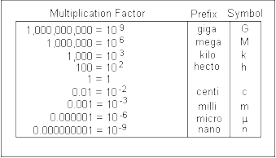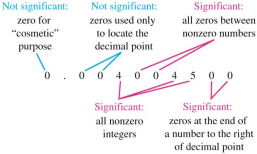## What is the smallest ratio of elements?Conversions

Ex) 355 km  10^3m  10^­3s = 355 m

S     1 km       ms              ms

Empirical Formula is the smallest ratio of elements.

Ex) C2 H3 O2 is empirical

C4 H6 O4 is not empirical. 2 can be divided out of them all.

Molecular formula is a multiple of the empirical formula.

Ex)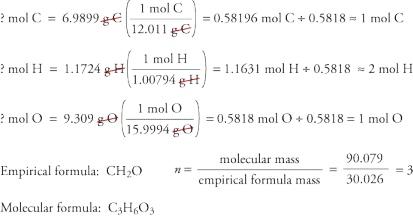Limiting reactant

## What is a multiple of the empirical formula?If you want to learn more check out What is the main cause of disease?

NH3 + O2         NO + H2O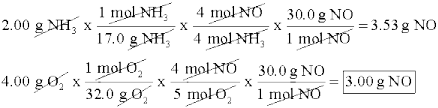NO is the limiting reactant.

Percent Yield

Actual yield          * 100%

Theoretical Yield

What is the percent yield of H2O if 138g is produced from 16g of H2 and excess O2? 2H2 + 02 = 2H2O

# g H2O = 16g H2 * 1 mol H2 * 2 mol H2O * 18.02g H20 = 143g H2O                                   2.02g H2    2 mol H2        1 mol H2O

% yield = actual * 100%  = 138g H2O *100% = 96.7%

theoretical              143g H2O  We also discuss several other topics like How can exchange theory be used to understand/interpret actors behavior?
If you want to learn more check out What are the five main points that make up dalton's atomic theory?

Formulas

Grams given * 1 mol * 6.022x10^23 molecules = ?molecules

Molar mass         1 mol

Of element(s)

XMass given * 1 mol of X     *   Ymoles given in formula   *  Grams of Y   = Grams of Y                   Molar mass of X        X moles given in formula       1 mol of Y

Molarity = Moles of solute              M = n           n = v * M      v = n

Volume of solute (L)                v                                       M

Molarity = mol          M = mol

L                      L

Electrolytes are compounds that ionize and conduct electricity

Strong acids and bases are strong electrolytes

Weak electrolytes are:

∙ HF

∙ HCN

∙ C2H4O2

Soluble Elements and compounds

∙ Li

∙ Na

∙ K

∙ Rb

∙ Cs

∙ NH4+ Don't forget about the age old question of How the behavior is acquired?

∙ NO3­

∙ ClO4­

∙ C2H3O2­

∙ SO4^2­  except Sr^2+ , Ba2+ , Hg2^2+ , Pb^2+ ∙ Cl­

∙ Br­           except Ag+ , Hg2^2+ , Pb^2+

∙ I

Oxidation: loss of one or more electrons

Reduction: gain of one or more electrons

Redox: electrons are transferred

H2 S O4     2(+1) + ? + 4(­2) = 0 net charge

+1  ?  ­2      S = +6

(charges)

Redox: hydrocarbon + 02(g)          CO2(g) + H2O(l)  Titration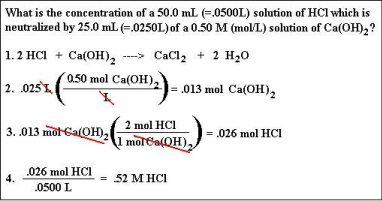Net Ionic Equation If you want to learn more check out What is the most effective treatment for panic disorder?
If you want to learn more check out When was the new world discovered?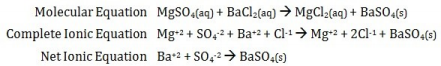Page ExpiredIt looks like your free minutes have expired! Lucky for you we have all the content you need, just sign up here